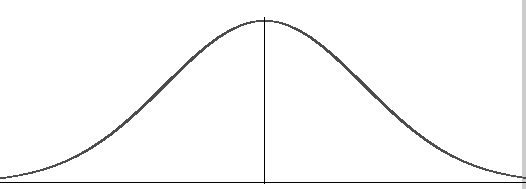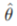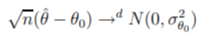# Asymptotic Normality

Share on

Asymptotic normality is a property of an estimator. “Asymptotic” refers to how an estimator behaves as the sample size gets larger (i.e. tends to infinity). “Normality” refers to the normal distribution, so an estimator that is asymptotically normal will have an approximately normal distribution as the sample size gets infinitely large.A normal distribution curve, sometimes called a bell curve, is one of the building blocks of a probabilistic model.

Asymptotic normality is very similar to the Central Limit Theorem. So similar in fact, that the two are (in general terms) the same thing. However, the CLT is a theorem, one that states:

The sampling distribution of the sample means approaches a normal distribution as the sample size gets larger—no matter what the shape of the population distribution.

Asymptotic normality is a property of converging weakly to a normal distribution.

## Formal Definition of Asymptotic Normality

An estimate(e.g. the sample mean) has asymptotic normality if it converges on an unknown parameter at a “fast enough” rate, 1 / √(n) (Panchenko, 2006).
Formally, an estimatehas asymptotic normality if the following equation holds:In statistics, we’re usually concerned with estimators. However, sequences and probability distributions can also show asymptotic normality. For example, a sequence of random variables, dependent on a sample size n is asymptotically normal if two sequences μn and σn exist such that:
limn>∞ P[(Tn – μn) / σn ≤ x] = φ(x)
Where “lim” is the limit (from calculus).
(Kolassa, 2014).

## References

DasGupta, A. (2008). Asymptotic Theory of Statistics and Probability (Springer Texts in Statistics). Springer.
Der Vaart, A. (2000). Asymptotic Statistics (Cambridge Series in Statistical and Probabilistic Mathematics). Cambridge University Press.
Kolassa, J. (2014). Asymptotic Normality. DOI: https://doi.org/10.1007/978-3-642-04898-2_125
Le Cam, L. (2000). Asymptotics in Statistics: Some Basic Concepts (Springer Series in Statistics) 2nd Edition. Springer.
Lehmann, E. (1998). Elements of Large-Sample Theory (Springer Texts in Statistics) Corrected Edition. Springer.
Panchenko, D. (2006). Lecture 3 Properties of MLE: consistency, asymptotic normality. Fisher information. Retrieved May 26, 2020 from: https://ocw.mit.edu/courses/mathematics/18-443-statistics-for-applications-fall-2006/lecture-notes/lecture3.pdf

CITE THIS AS:
Stephanie Glen. "Asymptotic Normality" From StatisticsHowTo.com: Elementary Statistics for the rest of us! https://www.statisticshowto.com/asymptotic-normality/
---------------------------------------------------------------------------Need help with a homework or test question? With Chegg Study, you can get step-by-step solutions to your questions from an expert in the field. Your first 30 minutes with a Chegg tutor is free!